# Reference Clock Distribution for a 325MHz IF Sampling System with over 30MHz Bandwidth, 64dB SNR and 80dB SFDR

Clock jitter introduced in an RF receiver through reference clock buffering and distribution can limit achievable system performance. The low jitter requirement is further exaggerated when a relatively high intermediate frequency (IF) is used in an effort to reap the benefits of relaxed front-end filter requirements. This article details the design of a 325MHz IF sampling system and introduces a clock buffer and distributor that converts a sine wave reference signal into a pair of differential LVPECL clocks appropriate to drive high speed ADCs, and does so while minimizing introduced jitter.

System Description

In an IF sampling (or undersampling) system, where the ADC performs the last stage of downconversion in an RF receiver, the higher the IF is, the less steep the image rejection filter in the RF front-end can be. This helps in reducing the filter cost, size and insertion loss, which further lessens the need for amplification, leading to even lower cost and power consumption. Figure 1 shows a typical RF receiver chain employing IF sampling.Figure 1. A typical single-IF-stage RF receiver block diagram.

The downside of designing a receiver with a relatively high IF is that system specifications become more susceptible to the degraded ADC performance while sampling a higher-frequency analog input signal. ADC spurious free dynamic range (SFDR), for instance, worsens with higher input frequencies. More importantly, the ADC aperture jitter and its clock jitter, combined, begin to define the achieved signal-to-noise ratio (SNR) while sampling faster inputs.

The effect of clock jitter can be demonstrated by comparing the voltage error magnitudes due to clock jitter while sampling two slewing signals, one with a higher slope than the other, using the same ADC and clock. This clock has the same amount of time jitter (tJ in s-RMS) while sampling the two signals as illustrated in Figure 2. The amount of uncertainty introduced due to clock jitter is indeed higher with the faster moving signal, and, hence, clock jitter is a major, if not dominant, error source limiting the SNR when the analog input has higher frequency content. Therefore, it is fundamental to keep the jitter of the ADC clock, denoted as the IF sampling clock in Figure 1, as low as possible.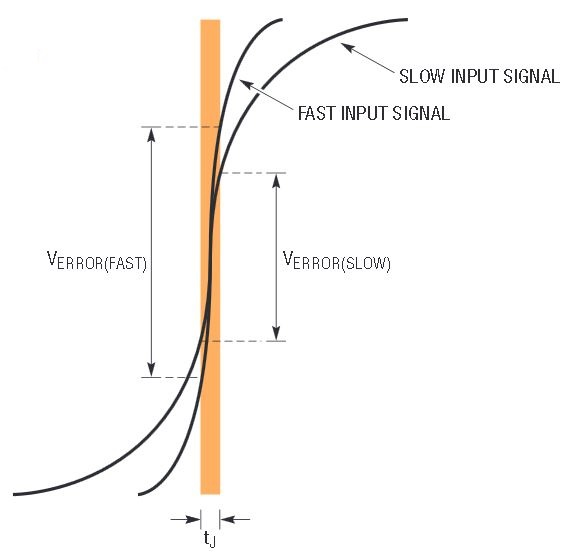Figure 2. Effect of clock jitter while digitizing slow and fast slewing signals.

To avoid AM-to-PM noise conversion at the ADC clock input, the clock should have a high slew rate, ideally be a square wave. The clock input of the ADC performs the role of a limiter, taking in a signal and squaring it by making decisions at the input signal’s zero (or some other reference) crossings. AM-to-PM noise conversion occurs when the incoming signal has a slow slew rate, like in a low frequency and/or amplitude sine wave, where the signal goes through the zero crossings what resembles slow motion as compared to a square wave. If there is any type of AM noise, for example, resistor thermal noise, coupled noise from the power supplies, etc., the zero crossings of the incoming signal become inconsistent between consequent edges, leading to the creation of jitter at the limiter’s output; thus, AM noise is converted into PM noise. Whereas, and if the incoming signal rushes through the zero crossing, as an LVPECL signal would normally do due to its fast rise and fall times, the AM noise added to the clock has no or very little chance of being converted into PM noise.

Also, most modern ADCs require their clock input to be driven differentially to attain their optimal performance. The clock signals are commonly routed on the PCB for quite some distance since their source and destination are usually not located close to each other. Running the clock signals in differential form makes them immune to coupling and leads to an overall more robust design as compared to single-ended clock routing.

The LO signal shown in Figure 1 is typically generated from a phase-locked loop (PLL) system. The PLL requires a reference clock to lock the LO to. Traditionally, 10MHz had been a common reference frequency. However, much higher frequency reference clocks are becoming more widespread nowadays. As a matter of fact, 100MHz and higher frequencies are not uncommon in modern RF designs.

The reference clock is usually generated from an OCXO or a TCXO device, which typically has very low jitter (or phase noise). If the PLL reference clock’s frequency is chosen to be reasonably higher than twice the received RF channel’s bandwidth (or that of multiple channels in a receiver where two or more adjacent channels are digitized simultaneously), the same reference signal can also be used to clock the IF sampling ADC, following some proper frequency planning. Ideally, the IF selectivity filter’s passband and the majority of its transition zone should fit into a single Nyquist zone of the ADC to avoid frequency folding. This point is clarified with the help of the IF filter amplitude response given in Figure 3, where the IF is chosen to match the 7th Nyquist zone of the ADC. In Figure 3, fS stands for the sampling rate of the ADC. In this case, the LO in Figure 1 would be chosen such that the down-converted signal output of the mixer is centered in the middle of the IF selectivity filter shown in Figure 3.Figure 3. An example of an IF filter amplitude response with respect to ADC sampling rate that avoids frequency folding.

Figure 4 summarizes the clock distribution scheme discussed above assuming a reference frequency of 100MHz. The clock buffer and distributor of Figure 4 has a very important role in this system as it receives a single-ended sine wave from the OCXO or TCXO device, and delivers two differential LVPECL signals suitable for routing to the ADC and the PLL. It should do so while adding a minimum amount of jitter to the distributed clock. The LTC6957-1 is a low additive jitter dual LVPECL output clock buffer that suits this application and meets all the requirements set forth in the discussion above. Other output formats can be achieved by employing different versions of the LTC6957. The LTC6957-2 has LVDS outputs and the LTC6957-3 and LTC6957-4 offer CMOS outputs.Figure 4. The reference clock distribution scheme.

Circuit Implementation

As already discussed, jitter is one of the main limiting factors for increasing the IF. To find out what type of a performance can be achieved using a common ADC along with the LTC6957-1 as the clock distributor, two Linear Technology demonstration circuits are modified and hooked up as shown in Figure 5.Figure 5. Schematic for the IF sampling system employing two Linear Technology demo boards with input 315.5MHz test tone and a 100MHz reference clock.

The LTC2153-14 is a 310Msps, 14-bit ADC that is well-specified for high analog input frequencies, making it suitable as the IF sampling ADC in this application. Its demonstration circuit, the DC1565A-G, is modified as shown in Figure 5.

The demonstration circuit, DC1765A-A, featuring the LTC6957-1, is used to buffer the sine wave output of a 100MHz OCXO. One of the DC1765A-A differential LVPECL output pairs is connected to the differential encode clock input of the DC1565A-G. The second pair could be used as the reference input of the LO generating PLL shown in Figure 1.

Given the ADC is clocked at 100MHz, the highest theoretical bandwidth that can be achieved, while avoiding aliasing, is 50MHz. As shown in Figure 3, the 7th Nyquist zone is picked, meaning that this 50MHz ideal bandwidth covers the 300MHz to 350 MHz frequency range. This would require an ideal brick-wall bandpass filter centered at 325MHz with a passband of 50MHz to pass only the IF information present in the 300MHz to 350 MHz range while rejecting everything else that could alias and interfere with the desired band.

Due to non infinitesimal transition zone between the filter passband and band-reject regions in a practical filter, besides center frequency tolerance, a more reasonable IF bandwidth selection in this case would be, for instance, a surface acoustic wave (SAW) filter with up to 30MHz bandwidth centered around 325MHz. SAW filters in this frequency range are becoming more readily available.

Calculation of the LTC6957-1 Additive Jitter

In a sampling system, jitter is usually measured through a 2-step process. The first step is to take a baseline SNR measurement with a relatively low frequency analog input tone at –1dBFS where jitter is not a major contributor of noise. Call this measurement SNR_BASE. A second measurement is taken using the same sampling clock source as in the first reading but with a higher-frequency analog input tone, still at –1dBFS. The SNR should degrade with the second measurement if the input frequency is high enough to realize jitter-related SNR degradation. Call this second measurement SNR_DEGRADED. It should be noted that in the second measurement, jitter can have multiple sources, including the sampling clock, the ADC aperture jitter and the analog input signal. Taking the RMS difference of the two measurements results in the jitter-limited SNR achieved at the higher input frequency had the ADC had no quantization or thermal noise at its analog input. Call this calculated number SNR_JTTR. These three terms are related as such: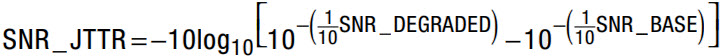The achieved SNR (SNR_JTTR) due to tJ amount of total jitter at the encode input of the ADC for an analog input tone at a frequency fIN is: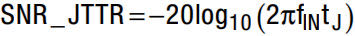Combining the last two equations and solving for tJ results in an equation that calculates the system jitter directly from the two measurement outcomes mentioned above.The LTC6957-1’s jitter contribution is measured following the procedure outlined above. Two sets of measurements are taken based on the schematic shown in Figure 5. The first measures the total intrinsic system jitter, which includes the ADC’s aperture jitter and these for the 100MHz and 315.5MHz sources but excluding the LTC6957-1. The second includes the LTC6957-1’s noise contribution. Taking the RMS difference between the two measurements results in the LTC6957-1’s additive jitter.

The total intrinsic system jitter excluding the contribution from the LTC6957-1 is found by connecting the 100MHz, 13dBm source straight into the encode input of the ADC with the use of a transformer to drive the clock input differentially. Two SNR measurements, excluding harmonics, are taken: one with a 10MHz, –1 dBFS sine wave at the analog input of the ADC, which reads 67.8dB. The second SNR measurement is taken with a 315.5MHz, –1 dBFS tone at the analog input of the ADC, resulting in 65.3 dB of SNR. The formula derived above calculates the total intrinsic system jitter: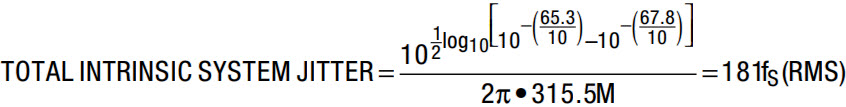The total system jitter after adding the LTC6957-1 to the system as shown in Figure 5 is found by taking another similar set of two measurements, one with an analog input of 10MHz and another with an analog input of 315.5MHz as described in the previous paragraph. The two SNR numbers are 67.8 dB and 64.24 dB, respectively. Using the same jitter formula as above results in the total system jitter: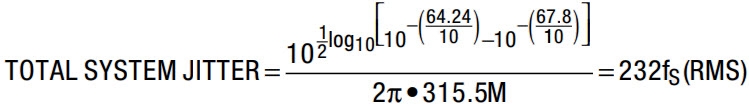Taking the RMS difference between the intrinsic and total system jitter numbers gives the additive jitter contribution of the LTC6957-1:Performance Summary

A 315.5MHz test tone is connected to the analog input of the modified DC1565A-G through a BPF that resembles the IF selectivity filter and an attenuator to dial the amplitude seen by the ADC to –1dBFS.

The DC1565A-G is connected via USB to a PC, where PScope1 data acquisition control software is used to look at two crucial parameters that affect the quality of the receiver: SNR and the SFDR. Figure 6 shows PScope in action, displaying a 131072-point FFT along with some analysis while having the 315.5MHz, –1dBFS tone as the analog input of the ADC and the 100MHz LVPECL signal buffered by the LTC6957-1 as the ADC encode clock. As can be seen in Figure 6, the achieved SNR is over 64dB and the SFDR is over 80dB. These are excellent numbers for a 325MHz IF sampler.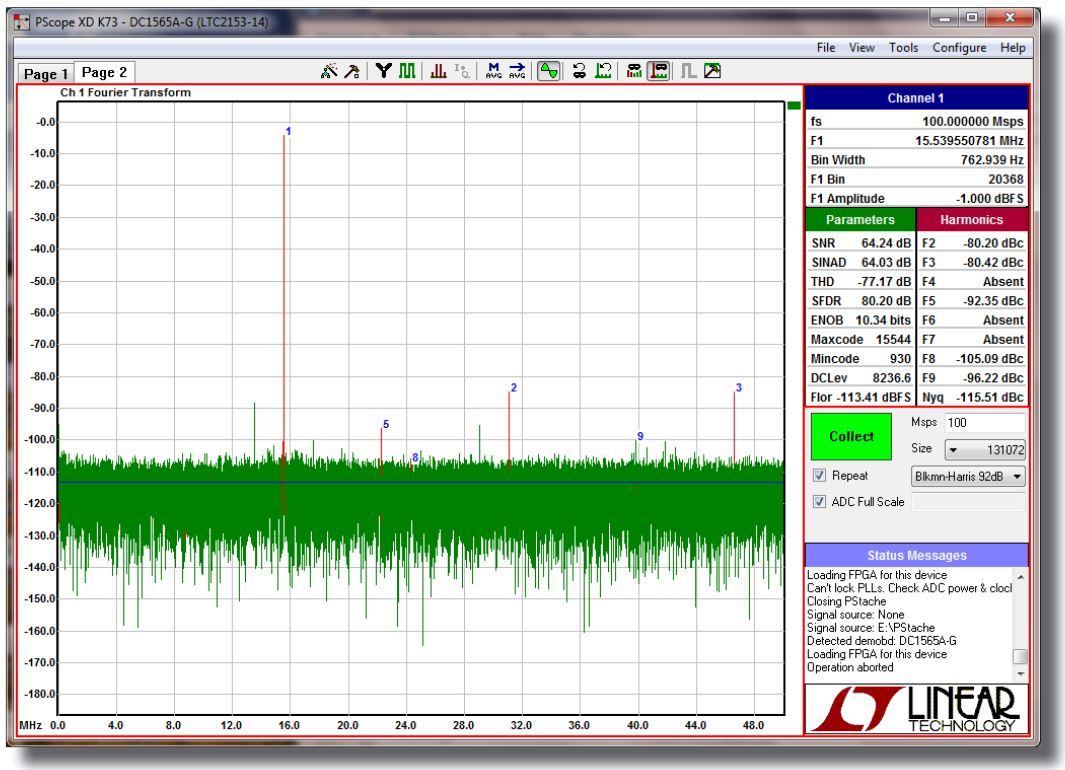Figure 6. Screenshot of PScope showing the FFT and achieved signal integrity parameters of the system shown in Figure 5.

Because the input of the LTC6957-1 is a 100MHz sine wave at +10dBm power into 50Ω, its internal bandwidth limiting filters (FILTA and FILTB), which help reduce the amount of added jitter when the input is low in amplitude and/or frequency, are both turned off per LTC6957 data sheet recommendation.

Conclusion

A 325MHz IF sampling system, as part of an RF receiver, is built and evaluated. The low jitter clock buffer and distributor, LTC6957-1, is employed to distribute the 100MHz system reference clock in LVPECL format to be used as the ADC sampling clock and the PLL reference. Performance of the IF sampling system is measured by looking at the SNR and SFDR numbers. An excellent 64dB of SNR and outstanding 80dB of SFDR are achieved with this system, enabling the relatively high IF sampling which helps relax the RF image rejection filter requirements.

Notes

1 PScope collects and analyzes data from the ADC in both time and frequency domains, and displays relevant parameters (available for download at ltspice.analog.com/software/ltcps.exe).